# Low degree minimal generators of phylogenetic semigroups

## Abstract

The phylogenetic semigroup on a graph is a set of edge labelings of the graph by non-negative integers. It generalizes the Jukes–Cantor binary model on trees. Minimal generating sets of phylogenetic semigroups have been described for trivalent trees by Buczyńska and Wiśniewski, and for trivalent graphs with first Betti number 1 by Buczyńska. We characterize the degree two minimal generators of the phylogenetic semigroup on any trivalent graph. Moreover, for any graph with first Betti number 1 and for any trivalent graph with first Betti number 2 we describe the minimal generating set of its phylogenetic semigroup.

## Introduction

Let $$G$$ be a graph. The phylogenetic semigroup on $$G$$ is a set of labelings of the edges of $$G$$ by non-negative integers fulfilling some additional conditions, see Sect. 2 for an explicit definition. This set has naturally the structure of a semigroup by edge-wise addition.

The phylogenetic semigroup on a trivalent graph was defined by Buczyńska  as a generalization of the affine semigroup of the Jukes–Cantor binary model on a trivalent tree. The Jukes–Cantor binary model is the simplest group-based model with the underlying group $$\mathbb {Z}_2$$. In , Buczyńska, Buczyński, Michałek and the author further generalized the definition of the phylogenetic semigroup to arbitrary graphs. This definition agrees with Buczyńska’s definition for trivalent graphs.

Besides phylogenetic algebraic geometry, phylogenetic semigroups appear in several other contexts. In , Jeffrey and Weitsman quantized the moduli space of flat $$\mathrm{SU }(2)$$ connections on a two-dimensional surface of genus $$g$$ using a real polarization. The dimension of the quantization is counted by integral fibers of the polarization, which are in one-to-one correspondence with the labelings of a trivalent graph $$G$$ with first Betti number $$g$$ that satisfy the quantum Clebsch–Gordan conditions. These labelings are exactly the elements of the phylogenetic semigroup on $$G$$. Moreover, the number of labelings that satisfy the quantum Clebsch–Gordan conditions matches the Verlinde formula for the $$\mathrm{SU }(2)$$ Wess–Zumino–Witten model in the quantum field theory .

In more recent work, Sturmfels and Xu  showed that the projective coordinate ring of the Jukes–Cantor binary model is a sagbi degeneration of the Cox ring of the blow-up of $$\mathbb {P}^{n+3}$$ at $$n$$ general points. Manon generalized their construction showing that the algebra of $$\mathrm{SL }_2(\mathbb {C})$$ conformal blocks for a stable curve of genus $$g$$ with $$n$$ marked points flatly degenerates to the semigroup algebra of the phylogenetic semigroup on a graph with first Betti number $$g$$ with $$n$$ leaves .

The minimal generators of low degree of phylogenetic semigroups have been previously studied for trees and graphs with first Betti number $$1$$. The phylogenetic semigroups on trees are generated by the degree one labelings, known as networks [4, 5]. Buczyńska studied the minimal generators of the phylogenetic semigroups on the trivalent graphs with first Betti number $$1$$. She proved that any minimal generator of the phylogenetic semigroup on a trivalent graph with first Betti number $$1$$ has degree at most two, and explicitly described the minimal generating sets .

We extend this result from trivalent graphs to general graphs: We describe the minimal generating set of the phylogenetic semigroup on any graph with first Betti number $$1$$. Moreover, we characterize the minimal generators of degree two of the phylogenetic semigroups on all trivalent graphs with first Betti number $$g>1$$.

We also specify the bound on the maximal degree of the minimal generating set for all graphs with first Betti number $$2$$. By , the maximal degree of the minimal generating set of the phylogenetic semigroup on a graph with first Betti number $$2$$ is at most three. We explicitly characterize when the maximal degree three is attained, and when the maximal degree is equal to two or one. If the degree three is attained, we describe the minimal generators of degree three.

Finally, we list the maximal degrees of the minimal generating sets of the phylogenetic semigroups on some graphs with first Betti number $$3, 4$$, or $$5$$. We speculate that the maximal degree depends on the separateness of the cycles of the graph. Having low maximal degree is especially interesting from the perspective of $${\mathrm {SL}}_2(\mathbb {C})$$ conformal block algebras as this ensures a low maximal degree for the minimal generators of these algebras, see .

In Sect. 2, we introduce basics about phylogenetic semigroups on graphs. In Sect. 3, we give a shortened proof of the theorem by Buczyńska about the minimal generators of the phylogenetic semigroups on the trivalent graphs with first Betti number $$1$$, and we generalize the statement to general graphs with first Betti number $$1$$. In Sect. 4, we characterize the minimal generators of degree two for any trivalent graph. In Sect. 5, we study the explicit maximal degree of the minimal generating set of the phylogenetic semigroup on a graph with first Betti number $$2$$, and in some cases describe the minimal generating sets of the phylogenetic semigroups on graphs with first Betti number $$2$$. In Sect. 6, we complete the description of the minimal generating sets of the phylogenetic semigroups on the trivalent graphs with first Betti number $$2$$. In the last section, we list examples of these maximal degrees for graphs with first Betti numbers $$3,4$$, and $$5$$.

## Phylogenetic semigroups

In this section, we define phylogenetic semigroups on graphs as in  and recall some basic properties about these semigroups.

### Definition 2.1

Let $$G$$ be a graph. A path in $$G$$ is a sequence of edges which connect a sequence of vertices. Moreover, we require the first and the last vertex to be either both leaves or equal. In the latter case, a path is called a cycle. A path with no repeated vertices, except maybe the first and the last one, is a simple path. A network is a disjoint union of simple paths. A cycle edge is an edge on a cycle of $$G$$. A cycle leg is an edge incident to a cycle edge, but is not a cycle edge. We denote the disjoint sum of graphs $$G_1$$ and $$G_2$$ by $$G_1\sqcup G_2$$. We denote by $$G^e$$ the graph obtained from $$G$$ by cutting an internal edge $$e$$. More specifically, cutting an internal edge $$e$$ means replacing $$e$$ by two leaf edges $$e_1$$ and $$e_2$$ where $$\partial _1(e_1)=\partial _1(e)$$ and $$\partial _1(e_2)=\partial _2(e)$$. Here $$\partial _1(e)$$ and $$\partial _2(e)$$ denote the endpoints of an edge $$e$$.

### Definition 2.2

The first Betti number of a graph is the minimal number of cuts that would make the graph into a tree.

### Definition 2.3

Let $$G$$ be a graph with edge set $$E$$ and inner vertex set $$I$$. Define lattices

\begin{aligned} L_G=\biggl \{\omega \in \mathbb {Z}^E:\sum _{v\in e}\omega _e \in 2\mathbb {Z}\, \mathrm{ for\,every }\, v\in I\biggr \} \end{aligned}

andtogether with the degree map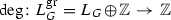given by the projection on the last summand.

### Definition 2.4

The lattice polytope associated with the Jukes–Cantor binary model on $$T$$ is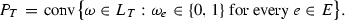An inequality description of this lattice polytope was suggested to us by an anonymous referee. On a claw tree, the inequality description is

\begin{aligned} \begin{aligned} \biggl \{\omega \in [0,1]^E:\sum _{e \in F} \omega _e - \!\!\sum _{e \in E \backslash F}\! \!\omega _e&\le |F|-1 \\&\quad \text { for all } F\subseteq E \text { of odd cardinality}\biggr \}. \end{aligned} \end{aligned}
(1)

This polytope is also known as the demicube or the halfcube. For a general tree $$T$$, we get the inequality description of $$P_T$$ by taking the union of inequalities in (1) for each internal vertex of $$T$$. Similarly, we can define $$P_G$$ for any graph, although the resulting polytope is in general not a lattice polytope. The cone over $$P_G$$ is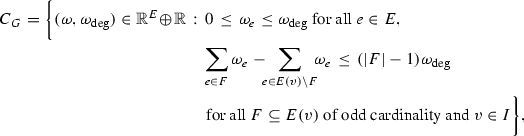where $$E(v)$$ denotes all edges incident to $$v$$. The phylogenetic semigroup $$\tau (G)$$ on $$G$$ is

\begin{aligned} \tau (G)=C_G\cap L_G^\mathrm{gr}. \end{aligned}

The phylogenetic semigroup $$\tau (G)$$ has a unique minimal generating set. We call the elements of the minimal generating set minimal generators, or sometimes also indecomposable elements of $$\tau (G)$$.

Definition 2.4 uses inequality description instead of point description for defining phylogenetic semigroups, but the phylogenetic semigroups we get are exactly the same as given by [3, Definition 2.5].

Now let $$G$$ be a trivalent graph and $$v$$ be an inner vertex of $$G$$. Let $$\{e_1, e_2, e_3\}$$ be the edges of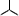and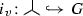be a map that is locally an embedding and sends the central vertex ofto $$v$$. For $$\omega \in \tau (G)$$ denote

\begin{aligned} a_v(\omega )=\omega _{i_v(e_1)}, \qquad b_v(\omega )=\omega _{i_v(e_2)}, \qquad c_v(\omega )=\omega _{i_v(e_3)}. \end{aligned}

In other words, $$a_v, b_v, c_v$$ measure the coefficients of $$\omega$$ at the edges incident to $$v$$.

Every element $$\omega \in \tau (G)$$ decomposes locally in a unique way into paths around an inner vertex $$v$$. This means that there exist non-negative integers $$x_v(\omega ), y_v(\omega ), z_v(\omega )$$ such that

\begin{aligned} a_v(\omega )=y_v(\omega )+z_v(\omega ),\qquad b_v(\omega )=x_v(\omega )+z_v(\omega ),\qquad c_v(\omega )=x_v(\omega )+y_v(\omega ), \end{aligned}

and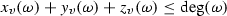, see also Fig. 1.

### Definition 2.5

The degree of $$\omega \in L_G^\mathrm{gr}$$ at an inner vertex $$v\in I$$ isFor trivalent graphs, Definition 2.4 becomes [2, Definition 2.18].

### Definition 2.6

([2, Definition 2.18]) For a trivalent graph $$G$$ the phylogenetic semigroup $$\tau (G)$$ on $$G$$ is the set of elements $$\omega$$ satisfying the following conditions:

Let $$G$$ be a graph and $$\overline{e}$$ an inner edge of $$G$$. Let $$e'$$ and $$e''$$ be the new leaf edges obtained by cutting $$G$$ at $$\overline{e}$$, as illustrated in Fig. 2. Then $$\omega \in \tau (G)$$ gives an element $$\overline{\omega } \in \tau (G^{\,\overline{e}})$$:

\begin{aligned} \overline{\omega }_e= {\left\{ \begin{array}{ll} \omega _e &{}\quad \mathrm{if }\quad e\notin \{e',e''\},\\ \omega _{\,\overline{e}} &{} \quad \mathrm{if }\quad e\in \{e',e''\}. \end{array}\right. } \end{aligned}

On the contrary, given $$\overline{\omega } \in \tau (G^{\,\overline{e}})$$, it gives an element $$\omega \in \tau (G)$$ if and only if $$\overline{\omega }_{e'}=\overline{\omega }_{e''}$$:

\begin{aligned} \omega _e={\left\{ \begin{array}{ll} \overline{\omega } _e &{}\quad \mathrm{if }\quad e\ne \overline{e},\\ \overline{\omega } _{e'} &{}\quad \mathrm{if }\quad e= \overline{e}. \end{array}\right. } \end{aligned}

In , a polygon graph $$G$$ was defined as a graph with $$2k$$ edges, $$k$$ of which form the only cycle of $$G$$ and the remaining $$k$$ edges are cycle legs. The use of polygon graphs simplifies the study of the phylogenetic semigroups on the trivalent graphs with first Betti number $$1$$. We generalize this definition to be able to simplify the study of phylogenetic semigroups on any graph.

### Definition 2.7

A graph $$G$$ with first Betti number $$g\ge 1$$ is called a multiple polygon graph if for no edge $$e$$ we can write $$G^e=G'\sqcup G''$$ with $$G'$$ or $$G''$$ a tree with more than one edge, see Fig. 3 for examples. A multiple polygon graph is a polygon graph if it has first Betti number $$1$$.

### Lemma 2.8

Given a graph $$G$$ with first Betti number $$g\ge 1$$, there exist non-cycle inner edges $$e_1,\ldots ,e_k$$ of $$G$$ such that $$G^{e_1,\ldots ,e_k}=G_0\sqcup G_1 \sqcup \dots \sqcup G_{k}$$ where $$G_0$$ is a multiple polygon graph and $$G_1,\ldots ,G_k$$ are trees.

### Proof

Choose all non-cycle edges $$e$$ such that we can write $$G^e=G'\sqcup G''$$ with $$G''$$ a tree with more than one edge and $$e$$ maximal with this property, i.e. there is an edge $$\overline{e}$$ incident to $$e$$ such that we cannot write $$G^{\overline{e}}={G}'\sqcup {G}''$$ with $$G'$$ or $$G''$$ a tree. $$\square$$

### Lemma 2.9

Let $$G$$ be a graph and $$\omega \in \tau (G)$$. Let $$e$$ be a non-cycle inner edge such that $$G^e=G'\sqcup G''$$ with $$G''$$ a tree. Then any decomposition of $$\omega |_{G'}\in \tau (G')$$ lifts to a decomposition of $$\omega \in \tau (G)$$.

### Proof

This lemma is stated for trivalent graphs in [2, Lemma 2.31]. Since $$\tau (T)$$ is generated in degree one for any tree $$T$$ [5, Proposition 18], then the proof works for the general case exactly the same way as it does for the trivalent case. $$\square$$

Applying Lemma 2.9 iteratively we get

### Corollary 2.10

Let $$G$$ be a graph and $$\omega \in \tau (G)$$. Let $$e_1,\ldots ,e_k$$ be non-cycle inner edges such that $$G^{e_1,\ldots ,e_k}=G_0\sqcup G_1 \sqcup \dots \sqcup G_{k}$$ where $$G_0$$ is a multiple polygon graph and $$G_1,\ldots ,G_k$$ are trees. Then any decomposition of $$\omega |_{G_0}\in \tau (G_0)$$ lifts to a decomposition of $$\omega \in \tau (G)$$.

## Graphs with first Betti number 1

In this section, we study minimal generating sets of phylogenetic semigroups on graphs with first Betti number $$1$$. Buczyńska did this for trivalent graphs . We give a shortened proof of her result, and as a corollary describe the minimal generating set of the phylogenetic semigroup on any graph with first Betti number $$1$$.

Let $$G$$ be a graph. Networks can be seen as the degree one elements of $$\tau (G)$$. We define $$\omega$$ corresponding to a network $$\Gamma$$ in the following way:

\begin{aligned} \omega _e= {\left\{ \begin{array}{ll} 1 &{} \mathrm{if}\,\,e\,\,\mathrm{belongs} \,\,\mathrm{to}\,\,\Gamma ,\\ 0 &{} \mathrm{otherwise}. \end{array}\right. } \end{aligned}

It follows from the definition of a network that the parity condition is fulfilled for $$\omega$$ at every inner vertex of $$G$$. Hence $$\omega \in \tau (G)$$. We will often use the notion network for the corresponding labeling $$\omega \in \tau (G)$$.

It has been shown for various classes of graphs that networks are in one-to-one correspondence with the degree one elements of a phylogenetic semigroup [4, Lemma 3.3], [2, Lemma 2.26]. For an arbitrary tree, this was stated in [3, Sect. 2], but no proof was given. We did not find proofs for arbitrary trees or graphs in the literature, and therefore will present them here.

### Lemma 3.1

Let $$T$$ be a tree. There is a one-to-one correspondence between the networks and the degree one elements of $$\tau (T)$$.

### Proof

We will prove the lemma by induction on the number of inner vertices of $$T$$.

Base case:   The statement of the lemma clearly holds for claw trees.

Induction step:   Let $$T$$ be a tree with $$n>1$$ inner vertices and $$\omega \in \tau (T)$$ a degree one labeling. If $$T$$ has more than one connected component, then by induction $$\omega$$ restricted to any connected component is a disjoint union of simple paths. Hence, the labeling $$\omega$$ is a disjoint union of simple paths.

If $$T$$ has one connected component, let $$e$$ be an inner edge, $$e_1,e_2$$ the new leaf edges obtained by cutting $$T$$ at $$e$$ and write $$T^e=T_1\sqcup T_2$$. Then $$\omega$$ restricted to either tree is a disjoint union of simple paths. If $$\omega _e=0$$, then $$\omega$$ is the disjoint union of exactly the same simple paths. If $$\omega _e=1$$, then the simple path of $$T_1$$ containing $$e_1$$ and the simple path of $$T_2$$ containing $$e_2$$ are combined to one simple path of $$T$$ containing $$e$$. Hence, the labeling $$\omega$$ is a disjoint union of paths. $$\square$$

### Lemma 3.2

Let $$G$$ be a graph. There is a one-to-one correspondence between the networks and the degree one elements of $$\tau (G)$$.

### Proof

We will prove the lemma by induction on the first Betti number $$g$$ of $$G$$.

Base case:   The statement of the lemma holds for trees by Lemma 3.1.

Induction step:   Let $$G$$ be a graph with first Betti number $$g \ge 1$$ and $$\omega \in \tau (G)$$ a degree one labeling. Let $$e$$ be a cycle edge of $$G$$ and $$e_1,e_2$$ the new leaf edges obtained by cutting $$G$$ at $$e$$. The graph $$G^e$$ has first Betti number $$g-1$$. Then $$\omega$$ gives $$\overline{\omega }\in G^e$$ that is a disjoint union of simple paths containing both $$e_1,e_2$$ or neither of them. If $$\omega _e=0$$, then $$\omega$$ is the disjoint union of exactly the same simple paths. If $$\omega _e=1$$, then there are two possibilities. Either there is a simple path in $$\overline{\omega }$$ with the first edge $$e_1$$ and the last edge $$e_2$$ which lifts to a cycle in $$\omega$$. Or there is a simple path in $$\overline{\omega }$$ with the first edge $$e'$$ and the last edge $$e_1$$, and another simple path in $$\overline{\omega }$$ with the first edge $$e_2$$ and the last edge $$e''$$ where $$e',e''$$ are leaf edges. These simple paths in $$\overline{\omega }$$ lift to a single simple path in $$\omega$$ with the first edge $$e$$ and the last edge $$e'$$ in $$G$$. $$\square$$

### Corollary 3.3

Let $$G$$ be a graph. All networks are included in the minimal generating set of $$\tau (G)$$.

### Proof

For any graded affine semigroup $$\mathbb {N}\fancyscript{A}$$, all minimal generators of degree one are included in the minimal generating set of $$\mathbb {N}\fancyscript{A}$$. $$\square$$

### Theorem 3.4

([2, Theorem 2.29]) Let $$G$$ be a trivalent graph with first Betti number $$1$$ and $$\omega \in \tau (G)$$. Then $$\omega$$ is a minimal generator of $$\tau (G)$$ if and only if it satisfies one of the following conditions:

• $$\omega$$ is a network, or

• $$\omega$$ has degree two, and satisfies the following three conditions:

1. (i)

$$\omega _e=1$$ for all cycle edges $$e$$,

2. (ii)

$$\omega _e=2$$ for an odd number of cycle legs,

3. (iii)

$$\omega _e=0$$ for the remaining cycle legs.

We give a shortened proof of this theorem. The following lemma will be an important part of it.

### Lemma 3.5

Let $$G$$ be a graph with first Betti number $$1$$. Let $$\omega \in \tau (G)$$ be of degree $$d$$. If there is a cycle edge $$e$$ with $$\omega _e=0$$ or $$\omega _e=d$$, then $$\omega$$ decomposes as a sum of degree one elements.

### Proof

Let $$e$$ be a cycle edge and $$e_1,e_2$$ the new leaf edges obtained by cutting $$G$$ at $$e$$. Notice that $$G^e$$ is a tree. Then $$\omega$$ gives $$\overline{\omega } \in \tau (G^e)$$ that decomposes into degree one elements $$\overline{\omega } = \overline{\omega _1}+\cdots +\overline{\omega _d}$$. Since $$(\overline{\omega _i})_{e_1}=(\overline{\omega _i})_{e_2}$$ for all $$i$$, the decomposition $$\overline{\omega } = \overline{\omega _1}+\cdots +\overline{\omega _d}$$ gives a decomposition $$\omega = \omega _1+\dots +\omega _d$$ of $$\omega \in \tau (G)$$. $$\square$$

Proof of Theorem 3.4  By Corollary 2.10 we can assume that $$G$$ is a trivalent polygon graph. First, we prove that any minimal generator of $$\tau (G)$$ has degree at most two. Let $$\omega \in \tau (G)$$ be of degree $$d$$. Let $$e$$ be a cycle edge and $$e_1,e_2$$ the new leaf edges obtained by cutting $$G$$ at $$e$$. Then $$\omega$$ gives $$\overline{\omega } \in \tau (G^e)$$ that decomposes as a sum of degree one elements $$\overline{\omega } = \overline{\omega _1}+\dots +\overline{\omega _d}$$. If $$(\overline{\omega _i})_{e_1}=(\overline{\omega _i})_{e_2}$$ then $$\overline{\omega _i}$$ gives an element $$\omega _i\in \tau (G)$$. Otherwise, there exists $$j$$ such that $$(\overline{\omega _i})_{e_1}=(\overline{\omega _j})_{e_2}$$ and $$(\overline{\omega _j})_{e_1}=(\overline{\omega _i})_{e_2}$$, because $$\overline{\omega }_{e_1}=\overline{\omega }_{e_2}$$. Thus $$\overline{\omega _i}+\overline{\omega _j}$$ gives a degree two element $$\omega _i+\omega _j \in \tau (G)$$.

The degree one elements of $$\tau (G)$$ are networks by Corollary 3.2. By Lemma 3.5, all degree two indecomposable elements $$\omega$$ have $$\omega _e=1$$ on all cycle edges $$e$$. Since $$G$$ is a trivalent graph, we have $$\omega _e\in \{0,2\}$$ for all cycle legs because of the parity condition. Assume $$\omega _e=2$$ for an even number of cycle legs $$e_1,\ldots , e_{2k}$$ in the clockwise order. Denote by $$P_i$$ the simple path starting at $$e_i$$ and ending at $$e_{i+1}$$ (at $$e_0$$ for $$i=2k$$). Then $$\omega$$ decomposes as the sum of networks $$P_1\cup P_3 \cup \dots \cup P_{2k-1}$$ and $$P_2 \cup P_4 \cup \dots \cup P_{2k}$$. Hence, for $$\omega$$ indecomposable, $$\omega _e=2$$ for an odd number of cycle legs.

Conversely, assume that $$\omega \in \tau (G)$$ has degree two and fulfills (i), (ii), and (iii). Suppose $$\omega =\omega _1 + \omega _2$$ where $$\omega _1,\omega _2$$ are networks. For all cycle legs $$e$$ with $$\omega _e=2$$, we have $$(\omega _i)_e=1$$, since $$(\omega _i)_e\le 1$$ for all edges $$e$$. Hence, we have $$(\omega _i)_e=1$$ for odd number of leaves of $$G$$. But this is contradiction to the fact that $$\omega _i$$ is a network. $$\square$$

### Remark 3.6

We know from [2, 3] that a minimal generator of the phylogenetic semigroup on a graph with first Betti number $$1$$ has degree at most two. We showed this above to give a simple and self-containing proof.

### Corollary 3.7

Let $$G$$ be a graph with first Betti number $$1$$ and $$\omega \in \tau (G)$$. Then $$\omega$$ is a minimal generator of $$\tau (G)$$ if and only if it satisfies one of the following conditions:

• $$\omega$$ is a network, or

• $$\omega$$ has degree two, and satisfies the following three conditions:

1. (i)

$$\omega _e=1$$ for all cycle edges $$e$$,

2. (ii)

$$\omega _e=2$$ for an odd number of cycle legs,

3. (iii)

$$\omega _e=0$$ for the remaining cycle legs.

### Proof

Let $$G'$$ be a trivalent graph constructed from $$G$$ in the following way: Replace all vertices $$v$$ with valency higher than three by two new vertices $$v'$$ and $$v''$$ together with a new edge between them, let two edges incident to $$v$$ be incident to $$v'$$ and the rest of the edges incident to $$v$$ be incident to $$v''$$. Moreover, if $$v$$ is on the cycle, let one cycle edge incident to $$v$$ be incident to $$v'$$ and let the other cycle edge incident to $$v$$ be incident to $$v''$$. This assures that we do not add any cycle legs. After a finite number of replacements we get a trivalent graph $$G'$$. By the proof of [3, Lemma 4.1], $$\tau (G)$$ is the coordinate projection of $$\tau (G')$$ that forgets coordinates corresponding to new edges. In particular, if $$\omega '\in \tau (G')$$ is decomposable, then its projection in $$\tau (G)$$ is also decomposable.

By , any minimal generator of $$\tau (G)$$ has degree at most two. The degree one elements are networks. We are left with describing the indecomposable elements of degree two of $$\tau (G)$$. A degree two indecomposable element $$\omega \in \tau (G)$$ is the coordinate projection of a degree two indecomposable element of $$\tau (G')$$. Since all cycle legs of $$G'$$ are also cycle legs of $$G$$, then by Theorem 3.4 the conditions (i), (ii), (iii) are fulfilled for $$\omega$$.

Conversely, assume that the conditions (i), (ii), (iii) are fulfilled. Suppose $$\omega =\omega _1 + \omega _2$$ where $$\omega _1,\omega _2$$ are networks. For all cycle legs $$e$$ with $$\omega _e=2$$, we have $$(\omega _i)_e=1$$, since $$(\omega _i)_e\le 1$$ for all edges $$e$$. Hence, we have $$(\omega _i)_e=1$$ for odd number of leaves of $$G'$$. But this is a contradiction to the fact that $$\omega _i$$ is a network. $$\square$$

## Minimal generators of degree two

In this section, we describe the degree two indecomposable labelings for any trivalent graph $$G$$.

### Lemma 4.1

Let $$G$$ be any graph and $$\omega \in \tau (G)$$ a degree two labeling. If there exists a cycle $$G'$$ of $$G$$ such that $$\omega _e=1$$ for all cycle edges $$e\in G'$$, $$\omega _e=2$$ for an odd number cycle legs $$e$$ of $$G'$$ and $$\omega _e=0$$ for the remaining cycle legs $$e\in G'$$, then the labeling $$\omega$$ is indecomposable.

### Proof

If $$\omega$$ decomposes, then a decomposition of $$\omega$$ restricts to a decomposition of $$\omega |_{G'}\in \tau (G')$$ where $$G'$$ is a cycle together with its cycle legs. Thus the statement follows from Corollary 3.7. $$\square$$

### Lemma 4.2

Let $$G$$ be a trivalent graph and $$\omega \in \tau (G)$$ a degree two labeling. The labeling $$\omega$$ is indecomposable if and only if there exists a cycle $$G'$$ of $$G$$ together with its cycle legs such that $$\omega |_{G'}\in \tau (G')$$ is indecomposable.

### Proof

One direction follows from Lemma 4.1. We show by induction on the first Betti number of $$G$$ that if $$\omega \in \tau (G)$$ is a degree two indecomposable labeling then there exists a cycle $$G'$$ together with its cycle legs such that $$\omega |_{G'}$$ is a degree two indecomposable labeling.

Base case:   If the first Betti number of $$G$$ is $$1$$, then the statement follows from Theorem 3.4.

Induction step:   Assume that the first Betti number of $$G$$ is $$g>1$$. If more than one connected component of $$G$$ contains a cycle, then there exists a connected component $$C$$ of $$G$$ containing a cycle such that $$\omega |_{C}\in \tau (C)$$ is an indecomposable element of degree two. Since the first Betti number of $$C$$ is less than $$g$$, we know by induction that there exists a cycle $$G'$$ of $$C$$ together with its cycle legs such that $$\omega |_{G'}\in \tau (G')$$ is an indecomposable element of degree two.

Otherwise all cycles of $$G$$ live in the same connected component of $$G$$. If $$\omega _e=1$$ for all cycle edges $$e$$, then by the parity condition $$\omega _e\in \{0,2\}$$ for all cycle legs $$e$$. In particular, none of the cycle legs is simultaneously a cycle edge and there exists a cycle leg $$e$$ that separates some cycles of $$G$$. Let $$e_1,e_2$$ be the new leaf edges obtained by cutting $$G$$ at $$e$$ and write $$G^e=G_1\sqcup G_2$$. Then $$\omega$$ gives $$\omega _1 \in \tau (G_1)$$ and $$\omega _2 \in \tau (G_2)$$ with at least one of them indecomposable, otherwise one could lift these decompositions to a decomposition of $$\omega$$. By induction, for $$i$$ with $$\omega _i$$ indecomposable, there exists a cycle $$G'$$ of $$G_i$$ together with its cycle legs such that $$\omega _i |_{G'}\in \tau (G')$$ is indecomposable. Thus $$\omega |_{G'} \in \tau (G')$$ is indecomposable.

If there exists a cycle edge $$e$$ with $$\omega _e\in \{0,2\}$$, then let $$e_1$$ and $$e_2$$ be the new leaf edges obtained by cutting $$G$$ at $$e$$. The labeling $$\omega \in \tau (G)$$ gives a labeling $$\overline{\omega }\in \tau (G^e)$$ that is indecomposable. Otherwise one could lift a decomposition $$\overline{\omega }=\overline{\omega }_1+\overline{\omega }_2$$ to a decomposition $$\omega =\omega _1+\omega _2$$, because $$(\overline{\omega _i})_{e_1}=(\overline{\omega _i})_{e_2}$$. The graph $$G^e$$ has first Betti number less than $$g$$. By induction, there exists a cycle $$G'$$ of $$G^e$$ together with cycle legs such that $$\overline{\omega }|_{G'}\in \tau (G')$$ is indecomposable. Thus $$\omega |_{G'} \in \tau (G')$$ is indecomposable. $$\square$$

## Graphs with first Betti number 2

We know from  that any minimal generator of the phylogenetic semigroup on a graph with first Betti number $$2$$ has degree at most three. In this section, we will explicitly describe which phylogenetic semigroups have which maximal degrees of the minimal generating sets for all graphs with first Betti number $$2$$. We will see that there are graphs with the maximal degrees of the minimal generators equal to one, two, and three. Our analysis is based on five different cases depending on the structure of the graph—whether the cycles live in different components of the graph, share at least one edge, share exactly a single vertex, there is a single edge connecting the cycles, or the cycles are more than one edge apart from each other, see Fig. 3 for the latter four cases.

### Remark 5.1

Assume a graph has a degree two vertex $$v$$. Denote the edges incident to $$v$$ by $$e_1$$ and $$e_2$$. By the definition of the phylogenetic semigroup on a graph, we have $$\omega _{e_1}=\omega _{e_2}$$ for $$\omega \in \tau (G)$$. Hence, the elements of $$\tau (G)$$ are in one-to-one correspondence with the elements of $$\tau (G')$$ where $$G'$$ is obtained from $$G$$ by replacing $$e_1$$ and $$e_2$$ by a single edge. To simplify future analysis, from now on we will assume that graphs possess no degree two vertices.

### Theorem 5.2

Let $$G$$ be a graph with first Betti number $$2$$. The maximal degree of a minimal generator of $$\tau (G)$$ is

• one if and only if $$G$$ does not contain any cycle legs that are not cycle edges;

• two if and only if

• the cycles of $$G$$ live in different connected components, or

• $$G$$ contains at least one cycle leg that is not a cycle edge, all cycles of $$G$$ live in the same connected component, and they are not separated by an inner vertex;

• three if and only if the minimal cycles of $$G$$ live in the same connected component and are more than one edge apart from each other.

We will study these different cases in Lemmas 5.55.10. As a corollary, we can describe the minimal generating sets of the phylogenetic semigroups on those trivalent graphs with first Betti number two that do not have any minimal generators of degree three.

From Theorem 5.2 and Lemma 3.2 we get

### Corollary 5.3

Let $$G$$ be a graph with first Betti number $$2$$ not containing any cycle legs that are not cycle edges. A labeling $$\omega \in \tau (G)$$ is a minimal generator of $$\tau (G)$$ if and only if $$\omega$$ is a network.

Besides, from Theorem 5.2 and Lemmas 3.24.2 we conclude

### Corollary 5.4

Let $$G$$ be a trivalent graph with first Betti number $$2$$, and

• the cycles of $$G$$ live in different connected components, or

• $$G$$ contains at least one cycle leg that is not a cycle edge, all cycles of $$G$$ live in the same connected component, and they are not separated by an inner vertex.

A labeling $$\omega \in \tau (G)$$ is a minimal generator of $$\tau (G)$$ if and only if it satisfies one of the following conditions:

• $$\omega$$ is a network, or

• $$\omega$$ has degree two and there exists a cycle $$G'$$ of $$G$$ together with its cycle legs such that $$\omega |_{G'}\in \tau (G')$$ is indecomposable.

### Lemma 5.5

Let $$G$$ be a graph with first Betti number $$2$$ that does not contain any cycle legs that are not cycle edges. The maximal degree of a minimal generator of $$\tau (G)$$ is one.

### Proof

The cycles of $$G$$ live in the same connected component. Otherwise $$G$$ would have a degree two vertex. If the connected component of $$G$$ containing the cycles has one vertex, then it is isomorphic to the right graph in Fig. 4. If the connected component of $$G$$ containing the cycles has two vertices, then it is isomorphic to the left graph in Fig. 4. The connected component of $$G$$ containing the cycles cannot have three or more vertices, because every vertex must belong to at least two cycles.

By computations with Normaliz , the phylogenetic semigroup on the left graph in Fig. 4 is

\begin{aligned} \mathbb {N} \{(0,0,0,1),(1,1,0,1),(1,0,1,1),(0,1,1,1)\}, \end{aligned}

where the last coordinate corresponds to the degree and the first three coordinates correspond to edges of $$G$$ in any fixed order.

By simple observation, the phylogenetic semigroup on the right graph in Fig. 4 is

\begin{aligned} \mathbb {N} \{(0,0,1),(1,0,1),(0,1,1)\}, \end{aligned}

where the last coordinate corresponds to the degree and the first two coordinates correspond to edges of $$G$$ in any fixed order. $$\square$$

### Lemma 5.6

Let $$G$$ be a graph with first Betti number $$2$$ and cycles living in different connected components. The maximal degree of a minimal generator of $$\tau (G)$$ is two.

### Proof

Define $$\omega \in \tau (G)$$ of degree two as follows: $$\omega _e=1$$ for all cycle edges $$e$$ of a cycle $$G'$$ of $$G$$, $$\omega _e=2$$ for one cycle leg of $$G'$$, and $$\omega _e=0$$ for all other cycle legs of $$G'$$. Extend this partial labeling of $$G$$ in any feasible way to a degree two labeling of $$G$$. By Lemma 4.1, $$\omega$$ is indecomposable. Hence, the maximal degree of a minimal generator of $$\tau (G)$$ is at least two.

On the other hand, we show that every element $$\omega \in \tau (G)$$ can be decomposed as a sum of degree one and degree two elements. By Corollary 3.7, $$\omega$$ restricted to each connected component decomposes as a sum of degree one and degree two elements. These decompositions can be combined to a decomposition of $$\omega \in \tau (G)$$ as a sum of degree one and degree two elements. Hence, the maximal degree of a minimal generator of $$\tau (G)$$ is exactly $$2$$. $$\square$$

Let $$T$$ be a trivalent tree and $$\omega _1,\omega _2\in \tau (T)$$ networks. Let $$v$$ be an inner vertex of $$T$$. Then either $$a_v(\omega _1)=a_v(\omega _2)$$, $$b_v(\omega _1)=b_v(\omega _2)$$, or $$c_v(\omega _1)=c_v(\omega _2)$$, since $$a_v(\omega _i)+b_v(\omega _i)+c_v(\omega _i)\in \{0,2\}$$ for $$i=1,2$$. We denote this edge by $$e$$. By exchanging values of $$\omega _1$$ and $$\omega _2$$ on all edges of $$T$$ that are on the same side with $$e$$ from $$v$$, we get $$\omega _1',\omega _2'\in \tau (T)$$ such that $$\omega _1+\omega _2=\omega _1'+\omega _2'$$. We call this operation branch swapping.

### Lemma 5.7

Let $$G$$ be a graph with first Betti number $$2$$ containing at least one cycle leg that is not a cycle edge and with two cycles sharing at least one edge. The maximal degree of a minimal generator of $$\tau (G)$$ is two.

### Proof

By Corollary 2.10, we can assume that $$G$$ is a multiple polygon graph. There is at least one cycle leg $$e'$$ of $$G$$ that is not a cycle edge for any of the cycles of $$G$$. Assume that $$e'$$ is a cycle leg of a cycle $$G'$$. Define $$\omega$$ of degree two as follows: $$\omega _e=1$$ for all cycle edges $$e$$ of $$G'$$, $$\omega _{e'}=2$$, and $$\omega _e=0$$ for all other edges $$e$$ of $$G$$. By Lemma 4.1, the labeling $$\omega \in \tau (G)$$ is indecomposable. Hence, the maximal degree of a minimal generator is at least two.

On the other hand, we show that every element $$\omega \in \tau (G)$$ can be decomposed as a sum of degree one and degree two elements. If $$G$$ is not trivalent, then by [3, Lemma 4.1] we can construct a trivalent graph $$G'$$ with first Betti number $$2$$ such that the maximal degree of the minimal generating set of $$\tau (G)$$ is less or equal than the one of $$\tau (G')$$. Moreover, two cycles of $$G'$$ share an edge. Hence, we can assume that $$G$$ is a trivalent graph.

If there is a cycle edge $$e$$ of $$G$$ with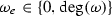, we construct the graph $$G^e$$ with first Betti number $$1$$ by cutting $$G$$ at $$e$$. Denote the new leaf edges by $$e_1$$ and $$e_2$$. The labeling $$\omega$$ gives a labeling $$\overline{\omega }$$ of $$G^e$$. By Theorem 3.4, the labeling $$\overline{\omega }$$ can be decomposed as a sum of degree one and two labelings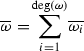where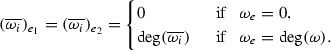Hence, the decomposition of $$\overline{\omega }$$ gives a decomposition of $$\omega$$ with all labelings having degree one or two. From now on we assume that there is no cycle edge $$e$$ of $$G$$ with.

There are exactly two vertices of $$G$$ incident to three cycle edges. We denote them by $$u$$ and $$v$$. We construct a tree $$G'$$ from $$G$$ by replacing the vertex $$u$$ with three new vertices $$u_1,u_2$$, and $$u_3$$ as in Fig. 5. The labeling $$\omega$$ gives a labeling $$\omega '$$ of $$G'$$. Abusing the notation slightly, we denote by $$a_u(\omega '),b_u(\omega '),c_u(\omega ')$$ the coordinates of $$\omega '$$ corresponding to leaf edges with endpoints $$u_1,u_2,u_3$$, respectively.

The labeling $$\omega '$$ can be decomposed as a sum of degree one labelingsFrom this we want to construct a decomposition of $$\omega \in \tau (G)$$. To lift an element of $$\tau (G')$$ to an element of $$\tau (G)$$, the parity and the degree condition have to be satisfied at leaf edges with endpoints $$u_1,u_2,u_3$$. This is not true for all $$\omega ' _i$$. We need to combine and alter these elements. We will use local paths to assure the parity and degree conditions are satisfied. We will construct the decomposition of $$\omega \in \tau (G)$$ iteratively. In each step, we construct a degree one or two element $$\omega ^*$$ and then replace $$\omega -\omega ^*$$ with $$\omega$$.

Case 1.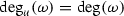.   Note that $$x_u(\omega ),y_u(\omega ),z_u(\omega )\ge 1$$, otherwise there would be a cycle edge $$e$$ of $$G$$ with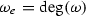.

• If there is $$\omega '_i$$ with exactly two of $$a_u(\omega '_i),b_u(\omega '_i),c_u(\omega '_i)$$ equal to 1, then $$\omega '_i$$ can be lifted to a degree one labeling of $$G$$.

• Otherwise if there is $$\omega '_i$$ with exactly one of $$a_u(\omega '_i),b_u(\omega '_i),c_u(\omega '_i)$$ equal to 1, then there is $$\omega '_j$$ with all of $$a_u(\omega '_j),b_u(\omega '_j),c_u(\omega '_j)$$ equal to 1. Then $$\omega '_i+\omega '_j$$ can be lifted to a degree two labeling of $$G$$.

• Otherwise there has to be $$\omega '_i$$ with all of $$a_u(\omega '_i),b_u(\omega '_i),c_u(\omega '_i)$$ equal to 0. Then there is $$\omega '_j$$ with all of $$a_u(\omega '_j),b_u(\omega '_j),c_u(\omega '_j)$$ equal to 1. After branch swapping of $$\omega '_i$$ and $$\omega '_j$$ at $$v$$, we get a labeling with exactly two values corresponding to $$a_u,b_u,c_u$$ equal to 1. It can be lifted to a degree on labeling of $$G$$.

Case 2.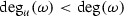.

• If there exists $$\omega ' _i$$ with $$a_u(\omega '_i)=b_u(\omega '_i)=c_u(\omega '_i)=0$$, then $$\omega ' _i$$ lifts to a labeling of $$\tau (G)$$.

Otherwise consider two subcases:

Case 2.1. $$x_u(\omega ),y_u(\omega ),z_u(\omega )\ge 1$$.

• If there is $$\omega '_i$$ with exactly two of $$a_u(\omega '_i),b_u(\omega '_i),c_u(\omega '_i)$$ equal to 1, then $$\omega '_i$$ can be lifted to a degree one labeling of $$G$$.

• Otherwise if there is $$\omega '_i$$ with all of $$a_u(\omega '_i),b_u(\omega '_i),c_u(\omega '_i)$$ equal to 1, then there is $$\omega ' _j$$ with exactly one of $$a_u(\omega '_j),b_u(\omega '_j),c_u(\omega '_j)$$ equal to 1. Then $$\omega '_i+\omega '_j$$ can be lifted to a degree two labeling of $$G$$.

• Otherwise all $$\omega '_i$$ have exactly one of $$a_u(\omega '_i),b_u(\omega '_i),c_u(\omega '_i)$$ equal to 1. Since $$x_u(\omega )\ge 1$$, there is $$\omega ' _i$$ with $$a_u(\omega ' _i)=c_u(\omega ' _i)=0$$ and $$b_u(\omega ' _i)=1$$, and $$\omega ' _j$$ with $$a_u(\omega ' _j)=b_u(\omega ' _j)=0$$ and $$c_u(\omega ' _j)=1$$. Then $$\omega ' _i+\omega ' _j$$ can be lifted to a degree two labeling for $$G$$.

Case 2.2. Exactly two of $$x_u(\omega ),y_u(\omega ),z_u(\omega )\ge 1$$.   It is not possible to have only one $$x_u(\omega ),y_u(\omega ),z_u(\omega )\ge 1$$, because we assumed $$\omega _e> 0$$ for every cycle edge $$e$$. We assume that $$x_u(\omega ),y_u(\omega )\ge 1$$, the other two cases are analogous.

• If there is $$\omega '_i$$ with exactly $$b_u(\omega '_i),c_u(\omega '_i)$$, or $$a_u(\omega '_i),c_u(\omega '_i)$$ equal to 1, then $$\omega '_i$$ can be lifted to a degree one labeling of $$G$$.

• Otherwise if there is $$\omega '_i$$ with exactly $$a_u(\omega '_i),b_u(\omega '_i)$$ equal to 1, there is $$\omega ' _j$$ with exactly $$c_u(\omega '_i)$$ equal to 1, since $$c_u(\omega )>a_u(\omega )$$ and $$c_u(\omega )>b_u(\omega )$$. After branch swapping $$\omega ' _i$$ and $$\omega ' _j$$ at $$v$$, we either get a labeling with all values corresponding to $$a_u,b_u,c_u$$ equal to 0 or a labeling with values corresponding to $$b_u,c_u$$ equal to 1 or a labeling with values corresponding to $$a_u,c_u$$ equal to 1. They all can be lifted to a degree one labeling of $$G$$.

• Otherwise if there is $$\omega '_i$$ with all of $$a_u(\omega '_i),b_u(\omega '_i),c_u(\omega '_i)$$ equal to 1, there is $$\omega ' _j$$ with exactly $$c_u(\omega '_j)$$ equal to 1, since $$c_u(\omega )>a_u(\omega )$$ and $$c_u(\omega )>b_u(\omega )$$. Then $$\omega _i+\omega _j$$ can be lifted to a degree two labeling of $$G$$.

• Otherwise all $$\omega '_i$$ have exactly one of $$a_u(\omega '_i),b_u(\omega '_i),c_u(\omega '_i)$$ equal to 1. Since $$x_u(\omega )\ge 1$$, there is $$\omega ' _i$$ with $$a_u(\omega ' _i)=c_u(\omega ' _i)=0$$ and $$b_u(\omega ' _i)=1$$, and $$\omega ' _j$$ with $$a_u(\omega ' _j)=b_u(\omega ' _j)=0$$ and $$c_u(\omega ' _j)=1$$. Then $$\omega ' _i+\omega ' _j$$ can be lifted to a degree two labeling for $$G$$.

At each step a degree one or two element is constructed. This assures that the iterative process comes to an end, because the degree of $$\omega$$ decreases. $$\square$$

### Lemma 5.8

Let $$G$$ be a graph with first Betti number $$2$$ containing at least one cycle leg that is not a cycle edge and with two cycles sharing exactly one vertex. The maximal degree of a minimal generator of $$\tau (G)$$ is two.

### Proof

By Corollary 2.10, we can assume that $$G$$ is a multiple polygon graph. There is at least one cycle leg $$e'$$ of $$G$$ that is not a cycle edge for any of the cycles of $$G$$. Assume that $$e'$$ is a cycle leg of a cycle $$G'$$. Define $$\omega$$ of degree two as follows: $$\omega _e=1$$ for all cycle edges $$e$$ of $$G'$$, $$\omega _{e'}=2$$, and $$\omega _e=0$$ for all other edges $$e$$ of $$G$$. By Lemma 4.1, the labeling $$\omega \in \tau (G)$$ is indecomposable. Hence, the maximal degree of a minimal generator is at least two.

On the other hand, we show that every element $$\omega \in \tau (G)$$ can be decomposed as a sum of degree one and degree two elements. We construct a trivalent graph $$G'$$ from $$G$$ as in [3, Lemma 4.1] such that the maximal degree of the minimal generating set of $$\tau (G)$$ is less than or equal to the one of $$\tau (G')$$. In particular, first we decrease the valency at the vertex $$v$$ that is on both cycles. We replace it by vertices $$v',v''$$, and an edge $$e$$ between them such that $$e$$ belongs to both cycles. We repeat replacing vertices until there are only trivalent vertices left. The graph $$G'$$ has two cycles that share at least one edge, thus we can apply Lemma 5.7. $$\square$$

### Lemma 5.9

Let $$G$$ be a graph with first Betti number $$2$$ and the two cycles separated by a single edge $$e$$. The maximal degree of a minimal generator of $$\tau (G)$$ is two.

### Proof

Define $$\omega \in \tau (G)$$ of degree two as follows: $$\omega _e=1$$ for all cycle edges $$e$$, $$\omega _e=2$$ for the single edge separating cycles, and $$\omega _e=0$$ for all other edges. By Lemma 4.1, $$\omega$$ is indecomposable. Hence, the maximal degree of a minimal generator of $$\tau (G)$$ is at least two.

On the other hand, we show that every element $$\omega \in \tau (G)$$ can be decomposed as a sum of degree one and degree two elements. If $$G$$ is not trivalent, then by [3, Lemma 4.1] we can construct a trivalent graph $$G'$$ with first Betti number $$2$$ such that the maximal degree of the minimal generating set of $$\tau (G)$$ is less than or equal to the maximal degree of the minimal generating set of $$\tau (G')$$. Moreover, we may assume that every time we replace a vertex $$v$$ on a cycle by vertices $$v',v''$$, and an edge between them, then $$v',v''$$ belong to the same cycle. This assures that the two cycles of $$G'$$ are separated by a single edge. Hence, we can assume that $$G$$ is a trivalent graph.

Let $$e_1,e_2$$ be the new leaf edges obtained by cutting $$G$$ at $$e$$, and write $$G^e=G_1\sqcup G_2$$. The labeling $$\omega$$ gives labelings $$\omega _1$$ of $$G_1$$ and $$\omega _2$$ of $$G_2$$. By Corollary 3.7, we can decompose $$\omega _1$$ and $$\omega _2$$ as a sum of degree one and degree two elements. Because all degree two labelings in these decompositions have values 0 or 2 corresponding to the edges $$e_1$$ and $$e_2$$, we can combine decompositions of $$\omega _1$$ and $$\omega _2$$ to get a decomposition of $$\omega$$ that consists of degree one and two elements. Hence, the maximal degree of a minimal generator of $$\tau (G)$$ is exactly two. $$\square$$

### Lemma 5.10

Let $$G$$ be a graph with first Betti number $$2$$ and the two cycles more than one edge apart from each other. The maximal degree of a minimal generator of $$\tau (G)$$ is three.

### Proof

By Corollary 2.10, we can assume that $$G$$ is a multiple polygon graph. We need to specify a degree three indecomposable element $$\omega \in \tau (G)$$. Fix an inner vertex $$v$$ on the path between the two cycles of $$G$$ and an edge $$e^*$$ incident to $$v$$ that is not on the path between the two cycles. Define $$\omega _e=2$$ for all cycle edges $$e$$ and all edges $$e$$ on the path between the cycles of $$G$$, $$\omega _{e^*}=2$$, and $$\omega _e=0$$ for all other edges $$e$$.

We will show that $$\omega$$ is indecomposable as a degree three labeling. By contradiction, assume $$\omega =\omega _1+\omega _2$$ where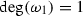and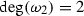. We must have $$(\omega _2)_e=1$$ for all cycle edges of $$G$$ and $$(\omega _2)_e=2$$ for both cycle legs $$e$$ that lie on the path between the two cycles. Hence, we have $$(\omega _2)_e=2$$ for all edges $$e$$ that lie on the path between the two cycles. Thus $$(\omega _1)_{e^*}=2$$. This leads to a contradiction, because. Hence, the labeling $$\omega$$ is a degree three indecomposable element in $$\tau (G)$$. $$\square$$

Lemmas 5.55.10 constitute Theorem 5.2.

## Minimal generators of degree three

In this section, we will describe the minimal generators of degree three of the phylogenetic semigroups on the trivalent graphs with first Betti number $$2$$ and with the cycles more than one edge apart from each other. As a result, we can describe the minimal generating sets of the phylogenetics semigroups on the trivalent graphs with first Betti number $$2$$ and with the cycles more than one edge apart from each other.

### Theorem 6.1

Let $$G$$ be a trivalent graph with first Betti number $$2$$ and the cycles of $$G$$ be more than one edge apart from each other. Then $$\omega \in \tau (G)$$ is a minimal generator of $$\tau (G)$$ if and only if it satisfies one of the following conditions:

• $$\omega$$ is a network, or

• $$\omega$$ has degree two, and there exists a cycle $$G'$$ of $$G$$ together with its cycle legs such that $$\omega |_{G'}\in \tau (G')$$ is indecomposable, or

• $$\omega$$ has degree three, and it satisfies the following three conditions:

1. (i)

$$\omega$$ restricted to any cycle with its cycle legs does not decompose as a sum of degree one labelings,

2. (ii)

$$\omega$$ restricted to an edge on the shortest path between two cycles has value one or two, and

3. (iii)

$$\omega$$ restricted to exactly one edge incident to an edge on the shortest path between two cycles that is not a cycle edge or an edge on the shortest path has value one or two, and has value zero or three on all other such edges.

Together with Corollaries 5.3 and 5.4, Theorem 6.1 completes the characterization of the minimal generating sets of the phylogenetic semigroups on the trivalent graphs with first Betti number $$2$$.

This section is organized as follows. In Lemma 6.2, we will characterize when a degree three labeling on a trivalent polygon graph cannot be decomposed as a sum of degree one labelings, and then we will extend this characterization to all trivalent graphs with first Betti number $$1$$ in Corollary 6.3. In Lemma 6.4, we will use these results to describe the indecomposable labelings of degree three on the trivalent graphs with first Betti number $$2$$ and cycles more than one edge apart from each other.

Let $$G$$ be a trivalent graph with first Betti number $$1$$ and $$\omega _2$$ a degree two indecomposable labeling. Label the cycle legs of $$G$$ where $$\omega _2$$ has value two by $$e_0,\ldots ,e_{2k}$$ in the clockwise order. Slightly abusing the notation, we write $$e_{i+j}$$ for $$e_{i+j \,\mathrm{mod}\, 2k+1}$$, where $$i+j>2k$$. We now consider $$\omega _2$$ as a union of paths without taking the degree into account. Label by $$P_{e'e''}$$ the simple path starting at a cycle leg $$e'$$ and going in the clockwise direction until reaching a cycle leg $$e''$$ . Write $$P_i$$ for $$P_{e_ie_{i+1}}$$. We say a cycle leg $$e$$ is between cycle legs $$e_i$$ and $$e_j$$, when $$e$$ is between cycle legs $$e_i$$ and $$e_j$$ in the clockwise direction.

### Lemma 6.2

Let $$G$$ be a trivalent polygon graph and $$\omega \in \tau (G)$$ a degree three labeling. Then $$\omega$$ cannot be decomposed as a sum of degree one labelings if and only if $$\omega =\omega _1+\omega _2$$ such that

•and,

• $$\omega _2$$ is indecomposable with value two on cycle legs $$e_0,\ldots ,e_{2k}$$ and value zero on all other cycle legs, and

• $$\omega _1$$ is up to a choice of the starting cycle edge $$e_0$$ one of the following unions of paths: $$P_0\cup P_2 \cup \dots \cup P_{2k-2}$$, $$P_0 \cup P_2 \cup \dots \cup P_{2k-4} \cup P_{e_{2k-2}e_{2k}}$$, or $$P_{0}\cup P_2 \cup \dots \cup P_{2k-2} \cup P_{e_{2k}e'}$$, where $$e'$$ is a cycle leg between $$e_{2k}$$ and $$e_{0}$$, or also the cycle path if $$k=0$$.

Moreover, then $$\omega$$ has a unique decomposition as $$\omega =\omega _1+\omega _2$$ withand.

### Proof

A degree three labeling $$\omega$$ can be always decomposed as $$\omega =\omega _1+\omega _2$$ withand. We show that unless $$\omega _1,\omega _2$$ are as in the statement of the lemma, we can alter $$\omega _1,\omega _2$$ to get $$\omega '_1,\omega '_2$$ such that $$\omega _1+\omega _2=\omega '_1+\omega '_2$$ and $$\omega '_2$$ decomposes as a sum of two degree one labelings.

If there is $$P_i$$ such that it does not intersect the network $$\omega _1$$, then the union of $$\omega _1$$ and $$P_i$$ is a network and the complement of $$P_i$$ in $$\omega _2$$ decomposes as the sum of $$P_{i+1}\cup P_{i+3} \cup \dots \cup P_{i-2}$$ and $$P_{i+2}\cup P_{i+4} \cup \dots \cup P_{i-1}$$. We will assume from now on that every $$P_i$$ intersects $$\omega _1$$.

If there exist $$e_i$$ and $$e_j$$ such that neither of them is incident to a path in $$\omega _1$$, then either $$e_j=e_{i+2l}$$ or $$e_i=e_{j+2l}$$ for some $$1\le l \le k$$. In the first case, let $$\Gamma$$ be the union of paths in $$\omega _1$$ from $$e_i$$ to $$e_j$$, and define $$\omega '_1=\omega _1 \backslash \Gamma \cup P_{i} \cup P_{i+2} \cup \dots \cup P_{j-2}$$ and $$\omega '_2=\omega _2 \backslash (P_{i} \cup P_{i+2} \cup \dots \cup P_{j-2}) \cup \Gamma$$. Then $$\omega '_2$$ decomposes as the sum $$\Gamma \cup P_{j} \cup P_{j+2} \cup \dots \cup P_{i-1}$$ and $$P_{j+1}\cup P_{j+3}\cup \dots \cup P_{i-2}\cup P_{i+1} \cup P_{i+3} \cup \dots \cup P_{j-1}$$. In the second case, the same discussion applies for $$i$$ and $$j$$ exchanged. We will assume from now on that there is at most one $$e_i$$ that is not incident to a path in $$\omega _1$$.

If $$\omega _1$$ corresponds to the cycle path $$P_{\mathrm{cycle }}$$ and $$k\ge 1$$, then $$\omega$$ decomposes as the sum of $$P_{e_2e_1}$$ and $$P_{e_0e_2}\cup P_3 \cup P_5 \cup \dots \cup P_{2k-1}$$ and $$P_{e_1e_3}\cup P_4 \cup P_6 \cup \dots \cup P_{2k}$$. Here we use that $$P_{\mathrm{cycle }}\cup P_0 \cup P_1 \cup P_2 = P_{e_0e_2} \cup P_{e_1e_3} \cup P_{e_2e_1}$$.

If there is a path $$P_{e'e''}$$ in $$\omega _1$$ such that not both $$e',e''$$ belong to $$\{e_0,\ldots ,e_{2k}\}$$, then we consider five different cases:

• If there is a path $$P_i$$ such that $$e',e''$$ are both between $$e_i$$ and $$e_{i+1}$$, define $$\omega _1'=\omega _1\backslash P_{e'e''}$$ and $$\omega _2'' =\omega _2\cup P_{e'e''}$$. Since $$P_{e'e''}\cup P_i=P_{e_ie''}\cup P_{e'e_{i+1}}$$, the labeling $$\omega '_2$$ decomposes as the sum of $$P_{e_ie''}\cup P_{i+1}\cup P_{i+3}\cup \dots \cup P_{i-2}$$ and $$P_{e'e_{i+1}}\cup P_{i+2}\cup P_{i+4}\cup \dots \cup P_{i-1}$$.

• If there is a path $$P_{i}$$ such that $$e'$$ is before $$e_i$$ and $$e''$$ is between $$e_{i}$$ and $$e_{i+1}$$, define $$\omega '_1=\omega _1\backslash P_{e'e''} \cup P_{e'e_i}$$ and $$\omega '_2=\omega _2 \backslash (P_{i-1}\cup P_i) \cup P_{e_{i-1}e_{i+1}} \cup P_{e_ie''}$$. Then $$\omega _1+\omega _2=\omega '_1+\omega '_2$$, since $$P_{e'e''}\cup P_{i-1}\cup P_i=P_{e'e_i}\cup P_{e_{i-1}e_{i+1}} \cup P_{e_ie''}$$. Then $$\omega '_2$$ decomposes as the sum of $$P_{e_{i-1}e_{i+1}}\cup P_{i+2}\cup \dots \cup P_{{i-3}}$$ and $$P_{e_ie''}\cup P_{i+1} \cup \dots \cup P_{i-2}$$.

• If there are paths $$P_{e_ie'}$$ and $$P_{e''e_{j}}$$ in $$\omega _1$$ such that $$e'$$ is between $$e_i$$ and $$e_{i+1}$$, and $$e''$$ is between $$e_{j-1}$$ and $$e_{j}$$, and $$e_j=e_{i+2l+1}$$ for some $$0\le l\le k-1$$, let $$\Gamma$$ be the union of paths between $$e_i$$ and $$e_j$$. Define $$\omega '_1=\omega _1\backslash \Gamma \cup P_i \cup P_{i+2}\cup \dots \cup P_{j-1}$$ and $$\omega '_2=\omega _2 \backslash (P_i \cup P_{i+2}\cup \dots \cup P_{j-1}) \cup \Gamma$$. Then $$\omega '_2$$ decomposes as the sum of $$P_{e_ie'}\cup P_{i+1} \cup P_{i+3} \cup \dots \cup P_{i-2}$$ and $$\Gamma \backslash P_{e_ie'} \cup P_{j+1} \cup P_{j+3} \cup \dots \cup P_{i-1}$$.

• If there are paths $$P_{e_ie'}$$ and $$P_{e''e_{j}}$$ in $$\omega _1$$ such that the edge $$e'$$ is between $$e_i$$ and $$e_{i+1}$$, the edge $$e''$$ is between $$e_{j-1}$$ and $$e_{j}$$ and $$e_j=e_{i+2l}$$ for some $$1\le l\le k$$, let $$\Gamma$$ be the union of paths between $$e_j$$ and $$e_i$$ together with $$P_{e_ie'}$$ and $$P_{e''e_{j}}$$. Define $$\omega '_1=\omega _1\backslash \Gamma \cup P_j \cup P_{j+2} \cup \dots \cup P_{i-1}$$ and $$\omega '_2=\omega _2\backslash (P_j \cup P_{j+2} \cup \dots \cup P_{i-1}) \cup \Gamma$$. Then $$\omega '_2$$ decomposes as the sum of $$\Gamma \backslash P_{e_ie'} \cup P_i \cup P_{i+2} \cup \dots \cup P_{j-2}$$ and $$P_{e_ie'}\cup P_{i+1} \cup P_{i+3} \cup \dots \cup P_{i-2}$$.

• If there are paths $$P_{e_ie'}$$ and $$P_{e_je''}$$ in $$\omega _1$$ such that the edge $$e'$$ is between $$e_i$$ and $$e_{i+1}$$, the edge $$e''$$ is between $$e_j$$ and $$e_{j+1}$$ and $$e_j=e_{i+2l}$$ for some $$1\le l\le k$$, let $$\Gamma$$ be the union of paths in $$\omega _1$$ between $$e_i$$ and $$e''$$ without $$P_{e_ie'}$$. Define $$\omega '_1=\omega _1 \backslash \Gamma \cup P_{i+1} \cup P_{i+3} \cup \dots \cup P_{j-1}$$ and $$\omega '_2=\omega _2\backslash (P_{i+1} \cup P_{i+3} \cup \dots \cup P_{j-1}) \cup \Gamma$$. Then $$\omega '_2$$ decomposes as the sum of $$P_{e_je''}\cup P_{j+1} \cup P_{j+3} \cup \dots \cup P_{j-2}$$ and $$P_{j} \cup P_{j+2} \cup \cdots \cup P_{i-1} \cup \Gamma \backslash P_{e_je''}$$. If $$e_j=e_{i+2l+1}$$ for some $$0\le l\le k-1$$ then the same discussion works for $$i$$ and $$j$$ exchanged.

If none of the five if-conditions holds, then the unique path of the form $$P_{e'e''}$$ in $$\omega _1$$ such that not both $$e',e''$$ belong to $$\{e_0,\ldots ,e_{2k}\}$$ must be $$P_{e_ie'}$$ or $$P_{e''e_{i+1}}$$ with $$e,'e''$$ between $$e_i$$ and $$e_{i+1}$$.

If there is a path in $$\omega _1$$ of the form $$P_{e_ie_j}$$, then we consider three different cases:

• If there is a path $$P_{e_ie_j}$$ in $$\omega _1$$ with $$e_j=e_{i+2l+1}$$ for $$1\le l \le k-1$$, then define $$\omega '_1=\omega _1\backslash P_{e_ie_j} \cup P_i \cup P_{i+2} \cup \dots \cup P_{j-1}$$ and $$\omega '_2=\omega _2\backslash (P_i \cup P_{i+2} \cup \dots \cup P_{j-1}) \cup P_{e_ie_j}$$. Since $$P_{e_ie_j}\cup P_{j-2}=P_{e_ie_{j-1}}\cup P_{e_{j-2}e_j}$$, then $$\omega '_2$$ decomposes as the sum of $$P_{e_ie_{j-1}}\cup P_j \cup P_{j+2} \cup \dots \cup P_{i-2}$$ and $$P_{i+1} \cup P_{i+3} \cup \dots \cup P_{j-4}\cup P_{e_{j-2}e_j}\cup P_{j+1} \cup P_{j+3} \cup \dots \cup P_{i-1}$$.

• If there is a path $$P_{e_ie_j}$$ in $$\omega _1$$ with $$e_j=e_{i+2l}$$ for $$2\le l \le k$$, assume that $$j=0$$. Define $$\omega '_1=\omega _1 \backslash P_{e_ie_j} \cup P_{e_ie_{j-2}} \cup P_{j-1}$$ and $$\omega '_2=\omega _2 \backslash (P_{j-3} \cup P_{j-2} \cup P_{j-1}) \cup P_{e_{j-3}e_{j-1}} \cup P_{e_{j-2}e_j}$$. Then $$\omega _1+\omega _2=\omega '_1+\omega '_2$$ since $$P_{e_ie_j}\cup P_{j-3}\cup P_{j-2} \cup P_{j-1}=P_{e_ie_{j-2}} \cup P_{e_{j-3}e_{j-1}} \cup P_{e_{j-2}e_j}\cup P_{j-1}$$. Then $$\omega '_2$$ decomposes as the sum of $$P_0 \cup P_2 \cup \dots \cup P_{e_{j-3}e_{j-1}}$$ and $$P_1 \cup P_3 \cup \dots \cup P_{e_{j-2}e_j}$$.

• If there are paths $$P_{e_{i_1}e_{i_2}},P_{e_{j_1}e_{j_2}}$$ in $$\omega _1$$ such that $$e_{i_2}=e_{i_1+2l}$$, $$e_{j_2}=e_{j_1+2m}$$ for $$1\le l,m \le k$$ and $$e_{j_2}=e_{i_1+2n+1}$$ for some $$0\le n\le k-1$$, assume that $$P_{e_{j_1}e_{j_2}}$$ is the next path with such property after $$P_{e_{i_1}e_{i_2}}$$ in the clockwise direction. Denote all paths between $$e_{i_1}$$ and $$e_{j_2}$$ in $$\omega _1$$ by $$\Gamma$$. Define $$\omega '_1=\omega _1 \backslash \Gamma \cup P_{i_1} \cup P_{i_1+2} \cup \dots \cup P_{j_2-1}$$ and $$\omega '_2=\omega _2 \backslash (P_{i_1} \cup P_{i_1+2} \cup \dots \cup P_{j_2-1}) \cup \Gamma$$. Then $$\omega '_2$$ decomposes as the sum of $$\Gamma \backslash P_{e_{j_1}e_{j_2}} \cup P_{j_1} \cup P_{j_1+2} \cup \cdots \cup P_{i_1-2}$$ and $$P_{i_1+1} \cup P_{i_1+3} \cup \dots \cup P_{j_1-2} \cup P_{e_{j_1}e_{j_2}} \cup P_{j_2+1} \cup \dots \cup P_{i_1-1}$$. If $$e_{j_2}=e_{i_1+2n}$$ for some $$1\le n\le k-1$$ then the same discussion works for $$i$$’s and $$j$$’s exchanged.

If none of the three if-conditions holds, then only paths in $$\omega _1$$ of the form $$P_{e_ie_j}$$ can be $$P_i$$, and at most one $$P_{e_je_{j+2}}$$.

Finally, we have to show that $$\omega _1$$ cannot simultaneously contain paths $$P_{e_ie'}$$ and $$P_{e_je_{j+2}}$$, where $$i,j\in \{0,\ldots ,2k\}$$ and $$e'$$ is between $$e_i$$ and $$e_{i+1}$$. We consider two different cases: $$e_j=e_{i+2l}$$ for $$1\le l \le k-1$$ and $$e_j=e_{i+2l+1}$$ for $$0\le l \le k-1$$. If we have $$P_{e'e_i}$$ instead of $$P_{e_ie'}$$ then we can apply the same discussion in the counterclockwise direction.

In the first case there must be $$e_t$$ with $$t=i+2l+1$$ between $$e'$$ and $$e_j$$ that is not incident to any of the paths in $$\omega _1$$. Otherwise paths between $$e'$$ and $$e_j$$ in $$\omega _1$$ would be $$P_{i+1},P_{i+3},\ldots ,P_{j-1}$$, which is not possible, since $$P_{e_je_{j+2}}$$ is in $$\omega _1$$. Let $$\Gamma$$ be the union of paths in $$\omega _1$$ between $$e_i$$ and $$e_t$$. Define $$\omega '_1=\omega _1 \backslash \Gamma \cup P_{i} \cup P_{i+2} \cup \dots \cup P_{t-1}$$ and $$\omega '_2=\omega _2 \backslash (P_{i} \cup P_{i+2} \cup \dots \cup P_{t-1}) \cup \Gamma$$. Then $$\omega '_2$$ decomposes as the sum of $$\Gamma \cup P_t \cup P_{t+2} \cup \dots \cup P_{i-2}$$ and $$P_{t+1}\cup P_{t+3} \cup \dots \cup P_{t-2}$$.

In the second case let $$\Gamma$$ be the union of paths in $$\omega _1$$ from $$e_i$$ to $$e_{j+2}$$. Define $$\omega '_1=\omega _1 \backslash \Gamma \cup P_i \cup P_{i+2} \cup \dots \cup P_{j+1}$$ and $$\omega '_2=\omega _2 \backslash (P_i \cup P_{i+2} \cup \dots \cup P_{j+1}) \cup \Gamma$$. Then $$\omega '_2$$ decomposes as the sum of $$P_{e_ie'}\cup P_{i+1} \cup P_{i+3} \cup \dots \cup P_{i-2}$$ and $$\Gamma \backslash P_{e_ie'} \cup P_{j+3} \cup P_{j+5} \cup \dots \cup P_{i-1}$$.

If none of the previous is true for $$\omega$$, then $$\omega$$ is as in the statement of the lemma. We will show that the only decomposition of $$\omega$$ is $$\omega =\omega _1+\omega _2$$. This implies that we cannot decompose $$\omega$$ as a sum of degree one labelings as $$\omega _2$$ is indecomposable.

In all four cases, we have $$\omega _e\ge 2$$ for a cycle leg $$e$$ if and only if $$e\in \{e_0,\ldots ,e_{2k}\}$$. Moreover, $$\omega _{\,\overline{e}}=2$$ holds for at most one $$\overline{e}\in \{e_0,\ldots ,e_{2k}\}$$. We construct a decomposition $$\omega =\omega '_1+\omega '_2$$ with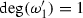and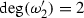. If $$\omega _1=P_{0}\cup P_2 \cup \dots \cup P_{2k-2} \cup P_{e_{2k}e'}$$, then $$(\omega '_2)_e=2$$ for all $$e\in \{e_0,\ldots ,e_{2k}\}$$ and $$(\omega '_2)_e=0$$ for all other cycle legs. Indeed, there is only one cycle leg $$e'$$ left with value one, but since the sum of values on all leaf edges must be even we have $$(\omega '_2)_{e'}=0$$. For the other three cases, $$(\omega '_2)_e=2$$ for all $$e\in \{e_0,\ldots ,e_{2k}\}\backslash {\overline{e}}$$, since $$\omega _e=3$$ for $$e\in \{e_0,\ldots ,e_{2k}\}\backslash {\overline{e}}$$. Thus also $$(\omega '_2)_{\overline{e}}=2$$, since the sum of values on all leaf edges must be even. It follows that $$(\omega '_2)_e=1$$ for all cycle edges, hence $$\omega '_i=\omega _i$$ for $$i\in \{ 1,2\}$$. $$\square$$

From Lemma 6.2 and Corollary 2.10 we get

### Corollary 6.3

Let $$G$$ be a trivalent graph with first Betti number $$1$$ and $$\omega \in \tau (G)$$ a degree three labeling. Then $$\omega$$ cannot be decomposed as a sum of degree one labelings if and only if $$\omega =\omega _1+\omega _2$$ such that

•and,

• $$\omega _2$$ is indecomposable with value two on cycle legs $$e_0,\ldots ,e_{2k}$$ and value zero on all other cycle legs, and

• $$\omega _1$$ restricted to the unique cycle with its cycle legs is up to a choice of the starting cycle edge $$e_0$$ one of the following unions of paths: $$P_0\cup P_2 \cup \dots \cup P_{2k-2}$$, $$P_0 \cup P_2 \cup \dots \cup P_{2k-4} \cup P_{e_{2k-2}e_{2k}}$$, or $$P_{0}\cup P_2 \cup \dots \cup P_{2k-4} \cup P_{e_{2k-2}e'}$$ where $$e'$$ is a cycle leg between $$e_{2k-2}$$ and $$e_{2k-1}$$, or also the cycle path if $$k=0$$.

### Lemma 6.4

Let $$G$$ be a trivalent graph with first Betti number $$2$$ and the cycles of $$G$$ be more than one edge apart from each other. A labeling $$\omega \in \tau (G)$$ of degree three is indecomposable if and only if the following conditions are fulfilled:

1. (i)

$$\omega$$ restricted to any cycle with its cycle legs does not decompose as a sum of degree one labelings,

2. (ii)

$$\omega$$ restricted to an edge on the shortest path between two cycles has value one or two, and

3. (iii)

$$\omega$$ restricted to exactly one edge incident to an edge on the shortest path between two cycles that is not a cycle edge or an edge on the shortest path has value one or two, and has value zero or three on all other such edges.

### Proof

By Corollary 2.10, we can assume that $$G$$ is a multiple polygon graph. Depict the edges on the shortest path between the two cycles horizontally and edges incident to them vertically below them as in Fig. 3d.

Assume $$\omega$$ restricted to a cycle $$G_1$$ together with its cycle legs decomposes as a sum of degree one elements. Let $$e$$ be a cycle leg of $$G_1$$ on the shortest path between $$G_1$$ and the other cycle. Write $$G^e=G_1\sqcup G_2$$. Then $$\omega$$ decomposes on $$G_2$$, and this decomposition can be extended to $$G$$.

Assume there is an edge $$\overline{e}$$ on the shortest path between two cycles of $$G$$ such that $$\omega _{\overline{e}}\in \{0,3\}$$. Let $$e',e''$$ be the new leaf edges obtained by cutting $$G$$ at $$e$$, and write $$G^{\overline{e}}=G'\sqcup G''$$. Then $$\omega |_{G'}$$ and $$\omega |_{G''}$$ can be decomposed as

\begin{aligned} \omega |_{G'}=\omega '_1+\omega '_2\qquad \mathrm{and }\qquad \omega |_{G''}=\omega ''_1+\omega ''_2 \end{aligned}

with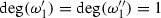and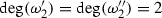. Furthermore, $$(\omega '_i)_{e'}=(\omega ''_i)_{e''}$$ for $$i=1,2$$ and hence they can be combined to a decomposition of $$\omega$$.

Assume now that the conditions (i) and (ii) are fulfilled. The labeling $$\omega$$ can be decomposed if and only if it can be decomposed as

\begin{aligned} \omega =\omega _1+\omega _2 \end{aligned}

withand. There is a unique way of defining $$\omega _1$$ and $$\omega _2$$ on cycles and cycle legs by Lemma 6.2. We try to construct a decomposition of $$\omega$$ on all other edges step-by-step going from left to right such that the decomposition is compatible with the decomposition on the cycle legs on the shortest path between the two cycles, and study when there exists no such decomposition. Let $$e$$ be the leftmost edge of the shortest path between two cycles where $$\omega _1$$ and $$\omega _2$$ are defined, and let the vertex $$v$$ be the right endpoint of $$e$$. We want to define $$b_v(\omega _i)$$ and $$c_v(\omega _i)$$ given $$a_v(\omega _i)$$ for $$i=1,2$$.

All possible local decompositions of $$\omega$$ at an inner vertex between the two cycles (assuming that horizontal edges have values one or two) are presented in Figs. 6 and 7. In Fig. 6 the value of $$\omega$$ at the vertical edge is zero or three. In Fig. 7 the value of $$\omega$$ at the vertical edge is one or two.

Given a local decomposition at $$v$$ as in Fig. 6 and $$a_v(\omega _i)$$, then there is a unique way of defining $$b_v(\omega _i)$$ and $$c_v(\omega _i)$$. In particular, if $$a_v(\omega _2)\in \{0,2\}$$ then $$b_v(\omega _2)\in \{0,2\}$$. If $$a_v(\omega _2)=1$$ then $$b_v(\omega _2)=1$$. Given a local decomposition at $$v$$ as in Fig. 7 and $$a_v(\omega _i)$$, then there might be a unique way of defining $$b_v(\omega _i)$$ and $$c_v(\omega _i)$$, or not, depending on the value of $$a_v(\omega _2)$$. If $$a_v(\omega _2)\in \{0,2\}$$ then $$b_v(\omega _2)=1$$. If $$a_v(\omega _2)=1$$ then one can define either $$b_v(\omega _2)\in \{0,2\}$$ or $$b_v(\omega _2)=1$$.

Let $$e$$ be a cycle leg that is on the path between two cycles. If $$\omega _{e}=2$$, then $$(\omega _1)_{e}=0$$ and $$(\omega _2)_{e}=2$$, because a degree two indecomposable element on a cycle can have only values zero and two on cycle legs by Theorem 3.4. If $$\omega _{e}=1$$, then $$(\omega _{e})_1=1$$ and $$(\omega _{e})_2=0$$ for the same reasons. Denote by $$e_\mathrm{r}$$ the cycle leg of the right cycle that are on the path between two cycles.

If the horizontal path contains labelings only as in Fig. 6, then $$b_v(\omega _2)\in \{0,2\}$$ for every vertex $$v$$ on the horizontal path. In particular, $$(\omega _2)_{e_\mathrm{r}}\in \{0,2\}$$, hence there exists a decomposition of $$\omega$$.

If at more than one vertex the local decomposition is as in Fig. 7, denote the first such vertex by $$v'$$ and the last one by $$v''$$. For all the vertices $$v$$ left from $$v'$$, the value $$b_v(\omega )\in \{0,2\}$$ is uniquely defined. For $$v'$$, we have $$b_{v'}(\omega )=1$$. For all the vertices $$v$$ between $$v'$$ and $$v''$$, we can define $$b_v(\omega )=1$$: If the local decomposition at $$v$$ is as in Fig. 7 then we have this choice by the discussion above. If the local decomposition at $$v$$ is as in Fig. 6 then $$b_v(\omega _2)=1$$ since $$a_v(\omega _2)=1$$ again by the discussion above. For $$v=v''$$, we define $$b_v(\omega _2)\in \{0,2\}$$. At all vertices $$v$$ to the right of $$v''$$, we have local decompositions as in Fig. 6, therefore $$b_v(\omega _2)\in \{0,2\}$$. In particular, $$(\omega _2)_{e_\mathrm{r}}\in \{0,2\}$$ and the decomposition of $$\omega$$ on the horizontal path is compatible with the decompositions of $$\omega$$ on both cycles. Hence, the labeling $$\omega$$ is decomposable.

On the other hand, if at one vertex $$v'$$ the local decomposition is as in Fig. 7 and at all other vertices the local decomposition is as in Fig. 6, then $$b_v(\omega _2)\in \{0,2\}$$ for all vertices $$v$$ left from $$v'$$ and $$b_v(\omega _2)=1$$ for all vertices $$v$$ to the right of $$v'$$ including $$v'$$ itself. In particular, $$(\omega _2)_{e_\mathrm{r}}=1$$ which is not compatible with the values of $$\omega _2$$ on the right cycle. Since all steps have been uniquely determined, then $$\omega$$ cannot be decomposed. $$\square$$

Now Theorem 6.1 follows from Lemmas 3.24.2, and 6.4.

## Examples

For any natural number $$g$$, there exists a graph $$G$$ with first Betti number $$g$$ such that the maximal degree of a minimal generator of $$\tau (G)$$ is one. Let $$G$$ be the graph with first Betti number $$g$$ that has two vertices and $$g+1$$ edges between the two vertices, as illustrated in Fig. 8. Then $$\tau (G)$$ is generated in degree one. By cutting all edges of $$G$$, we get two claw trees $$T',T''$$ with $$g+1$$ leaves. Let $$\omega \in \tau (G)$$ be a degree $$d$$ labeling. Then $$\omega$$ gives $$\omega '\in T'$$ and $$\omega '' \in T''$$ with $$\omega '=\omega ''$$ that we can decompose as a sum of $$d$$ degree one labelings exactly the same way on both trees. Gluing the decompositions of $$\omega '$$ and $$\omega ''$$ gives a decomposition of $$\omega$$ as a sum of degree one labelings.

Figures 910 and 11 present some examples of graphs with first Betti number $$3, 4$$, and $$5$$ together with the maximal degree of the minimal generating set of their phylogenetic semigroup.Footnote 1 Maximal degrees have been computed with Normaliz .

We note that the maximal degree tends to depend on the “separateness” of the cycles, exactly as we proved in Sect. 5 for the graphs with first Betti number $$2$$. This suggests that the maximal degree of the minimal generating set of the phylogenetic semigroup on the $$g$$-caterpillar graph is maximal among the graphs with first Betti number $$g$$ and that, for $$g$$ odd, there is no graph with first Betti number $$g$$ such that the maximal degree of its phylogenetic semigroup is $$g+1$$.

1. We thank Christopher Manon for introducing us the trivalent graph with first Betti number $$4$$ and maximal degree one, see Fig. 10.

## References

1. Bruns, W., Ichim, B.: Normaliz. http://www.home.uni-osnabrueck.de/wbruns/normaliz/

2. Buczyńska, W.: Phylogenetic toric varieties on graphs. J. Algebr. Comb. 35(3), 421–460 (2012)

3. Buczyńska, W., Buczyński, J., Kubjas, K., Michałek, M.: On the graph labellings arising from phylogenetics. Cent. Eur. J. Math. 11(9), 1577–1592 (2013)

4. Buczyńska, W., Wiśniewski, J.A.: On geometry of binary symmetric models of phylogenetic trees. J. Eur. Math. Soc. 9(3), 609–635 (2007)

5. Donten-Bury, M., Michałek, M.: Phylogenetic invariants for group-based models. J. Algebr. Stat. 3, 44–63 (2012)

6. Jeffrey, L.C., Weitsman, J.: Bohr-Sommerfeld orbits in the moduli space of flat connections and the Verlinde dimension formula. Commun. Math. Phys. 150(3), 593–630 (1992)

7. Manon, C.A.: The algebra of conformal blocks. arXiv:0910.0577

8. Sturmfels, B., Xu, Z.: Sagbi bases of Cox-Nagata rings. J. Eur. Math. Soc. 12(2), 429–459 (2010)

9. Verlinde, E.: Fusion rules and modular transformations in 2D conformal field theory. Nucl. Phys. B 300(3), 360–376 (1988)

## Acknowledgments

We thank Jaroslaw Buczyński, Christopher Manon, Henning Meyer, and Raman Sanyal for helpful conversations and comments. We thank an anonymous referee for pointing out the inequality description of a phylogenetic semigroup.

## Author information

Authors

### Corresponding author

Correspondence to Kaie Kubjas.